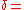xFeigenbaum constantsEncyclopedia

The Feigenbaum constants are two mathematical constant
Mathematical constant
A mathematical constant is a special number, usually a real number, that is "significantly interesting in some way". Constants arise in many different areas of mathematics, with constants such as and occurring in such diverse contexts as geometry, number theory and calculus.What it means for a...

s named after the mathematician Mitchell Feigenbaum
Mitchell Feigenbaum
Mitchell Jay Feigenbaum is a mathematical physicist whose pioneering studies in chaos theory led to the discovery of the Feigenbaum constants.- Biography :...

. Both express ratios in a bifurcation diagram
Bifurcation diagram
In mathematics, particularly in dynamical systems, a bifurcation diagram shows the possible long-term values of a system as a function of a bifurcation parameter in the system...

.

The first Feigenbaum constant ,4.66920160910299067185320382...

is the limiting ratio
Ratio
In mathematics, a ratio is a relationship between two numbers of the same kind , usually expressed as "a to b" or a:b, sometimes expressed arithmetically as a dimensionless quotient of the two which explicitly indicates how many times the first number contains the second In mathematics, a ratio is...

of each bifurcation interval to the next, or between the diameters of successive circles on the real axis of the Mandelbrot set
Mandelbrot set
The Mandelbrot set is a particular mathematical set of points, whose boundary generates a distinctive and easily recognisable two-dimensional fractal shape...

. Feigenbaum originally related this number to the period-doubling bifurcations in the logistic map
Logistic map
The logistic map is a polynomial mapping of degree 2, often cited as an archetypal example of how complex, chaotic behaviour can arise from very simple non-linear dynamical equations...

, but also showed it to hold for all one-dimensional maps with a single quadratic maximum. As a consequence of this generality, every chaotic system that corresponds to this description will bifurcate at the same rate. It was discovered in 1975.

The second Feigenbaum constant ,2.502907875095892822283902873218...,

is the ratio between the width of a tine and the width of one of its two subtines (except the tine closest to the fold).

These numbers apply to a large class of dynamical system
Dynamical system
A dynamical system is a concept in mathematics where a fixed rule describes the time dependence of a point in a geometrical space. Examples include the mathematical models that describe the swinging of a clock pendulum, the flow of water in a pipe, and the number of fish each springtime in a...

s (for example, dripping faucets to population growth).

Both numbers are believed to be transcendental
Transcendental number
In mathematics, a transcendental number is a number that is not algebraic—that is, it is not a root of a non-constant polynomial equation with rational coefficients. The most prominent examples of transcendental numbers are π and e...

, although they have not been proven to be so.

A proof of the universality of the Feigenbaum constants was given by Mikhail Lyubich
Mikhail Lyubich
Mikhail Lyubich is Professor of Mathematics at Stony Brook University. He received the 2010 Jeffery–Williams Prize from Canadian Mathematical Society.-Notes:...

in the 1990s.